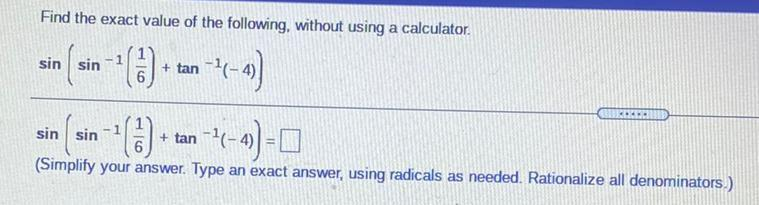Question:

# Find the exact value of the following, without using a calculator. 6 sin sin + tan -- ( 7-4) -- () + tan-2-4) = 0 . sin sin -1 (Find the exact value of the following, without using a calculator. 6 sin sin + tan -- ( 7-4) -- () + tan-2-4) = 0 . sin sin -1 (Simplify your answer. Type an exact answer, using radicals as needed. Rationalize all denominators.)